https://doi.org/10.5194/nhess-23-2607-2023
https://doi.org/10.5194/nhess-23-2607-2023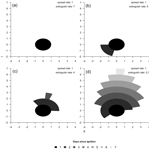# Estimating the effects of meteorology and land cover on fire growth in Peru using a novel difference equation model

Harry Podschwit, William Jolly, Ernesto Alvarado, Andrea Markos, Satyam Verma, Sebastian Barreto-Rivera, Catherine Tobón-Cruz, and Blanca Ponce-Vigo
Abstract

Statistical analyses of wildfire growth are rarely undertaken, particularly in South America. In this study, we describe a simple and intuitive difference equation model of wildfire growth that uses a spread parameter to control the radial speed of the modeled fire and an extinguish parameter to control the rate at which the burning perimeter becomes inactive. Using data from the GlobFire project, we estimate these two parameters for 1003 large, multi-day fires in Peru between 2001 and 2020. For four fire-prone ecoregions within Peru, a set of 24 generalized linear models are fit for each parameter that use fire danger indexes and land cover covariates. Akaike weights are used to identify the best-approximating model and quantify model uncertainty. We find that, in most cases, increased spread rates and extinguish rates are positively associated with fire danger indexes. When fire danger indexes are included in the models, the spread component is usually the best choice, but we also find instances when the fire weather index and burning index are selected. We also find that grassland cover is positively associated with spread rates and extinguish rates in tropical forests, and that anthropogenic cover is negatively associated with spread rates in xeric ecoregions. We explore potential applications of this model to wildfire risk assessment and burned area forecasting.

Share
Dates
1 Introduction

At least part of the reason that fire parameters such as spread and extinguish rates are overlooked by researchers is that the relevant data are uncommon. Daily fire growth data are rare, usually coming from case studies and administrative records , and although fire perimeter data are occasionally available , identifying which portions of the perimeter are active and inactive can be a difficult task . Where these data are available, they are subject to fairly high levels of uncertainty and often contain gaps and errors . These problems are particularly noticeable in locations that lack the historical records and technological resources that are more commonly found in the United States, Canada, and Australia. In South America for example, data regarding fire spread rates are available from only a few experimental studies , which may not be representative of the real-world conditions nor reliably be extrapolated to other locations. In most cases, information about real-world fire spread and extinguishment must be derived from satellite data .

In spite of these problems with data quantity and quality, we can use available research to develop some intuition regarding which environmental factors are likely to be relevant to Peruvian fire spread and extinguishment. Firefighter entrapments in the United States have been typically associated with rapid fire spread , and this fire parameter is both directly and indirectly associated with low fuel moisture and anomalously high wind speed. Consequently, a number of indexes have been proposed to estimate the risk of rapidly spreading fire based on the relevant atmospheric conditions. The energy release component (ERC) and burning index (BI) are commonly used in the United States to inform firefighting decisions and are calculated from a complex equation of temperature, precipitation, wind, humidity, cloud cover, fuel, topographic data, and geographic data . The spread component (SC) is derived from the ERC and BI, and provides a measure of idealized fire spread in certain fuel conditions . The fire weather index (FWI) was developed in the 1970s and is the preferred choice of fire danger index (FDI) in Canada and is structured somewhat similarly to the BI . The Keetch–Byram drought index (KBDI) measures the water balance of the upper soil layers and was developed to predict forest fire activity in the southeastern United States. Although developed for other regions, these FDIs can sometimes be informative of fire activity in other locations . Like FDIs, we can also develop some intuition about the effects of land cover on fire behavior using information from other locations. For instance, it is well known that vegetation can influence fire growth through sheltering of the flames from wind , and we might predict that forested land cover would have slower fire spread than non-forested land cover. It has also been shown that human presence, and by extension anthropogenic land cover types, can sometimes have an inhibitory effect on various fire parameters, although the relationship is complex and non-monotonic .

Existing conceptual climate–fire models can also help us predict how fuel availability and flammability are likely to mediate fire spread in novel environments. We call ecosystems that are frequently wet and have abundant fuel available (e.g., forests) climate-limited, and these ecosystems typically require exceptionally dry periods to permit large fire growth. On the other hand, we call ecosystems that are frequently dry and have low levels of fuel (e.g., grasslands) fuel-limited. Unlike climate-limited ecosystems, fuel-limited ecosystems require above-average antecedent precipitation to produce fuel loads of sufficient quantity and continuity to permit large fire growth . Indeed, naturally occurring large fires in the Amazon – an unambiguously climate-limited ecosystem – are very rare and require extremely low and sustained moisture to permit combustion (Cochrane2003). When fire does occur in the Amazon, it is usually intentionally set to clear forest for agriculture (Cochrane2003) and is characterized by low spread rates (≪1 m min−1) and low flame height (< 0.5 m) . In the Peruvian Andes – an ecoregion that is largely unforested – precipitation patterns follow a sawtooth pattern during peak fire years, where precipitation is anomalously high in the year previous, followed by dry weather during the peak fire year . Similarly, ENSO-related (El Niño–Southern Oscillation) increases in precipitation in the Sechura Desert of northern Peru are associated with increased fuel loads and subsequent increases in fire activity . Like conceptual models of fire activity, satellite-derived estimates of fire spread can also provide intuition regarding the scale and variability of fire spread in Peru. In South America, the typical individual fire is reported to increase in area at a rate of 0.5 km2 per day and increase radially at a rate of radial spread estimates of 0.7–0.8 km d−1. These growth estimates do not appear to vary strongly within South America, but large differences are found between xeric regions versus humid tropical regions globally .

Given that meteorology and land cover are known to be important influences on fire spread in other locations, we would like to explore the relevance of these factors to fire spread and fire extinguishment in Peru, where these relationships have not been well quantified. To do this, we will develop a simple difference equation model to summarize satellite-derived fire growth data. We will then build regression models to predict the difference equation parameters and determine if a suite of meteorological and land cover covariates are statistically significant predictors. The intended outcome of this analysis is to present a relatively simple method of predicting fire growth based on environmental conditions that can be applied nearly anywhere globally.

2 Model

The difference equation model begins with two simplifying assumptions regarding fire growth.

1. Fire spreads at a constant rate, r, from all angles from an ignition point.

2. After the first time step, a constant length, l, of the fire's perimeter is extinguished.

A graphical description of these dynamics for various values of r and l is shown below (Fig. 1). Note that in this study the word “growth” is reserved to describe changes in fire area and “spread” is reserved to describe radial changes in fire.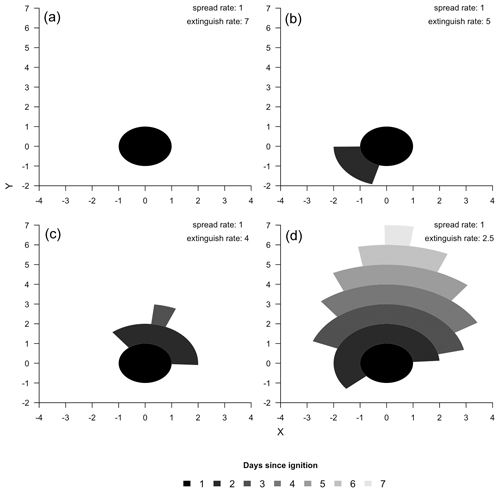Figure 1Graphical depiction of modeled fire growth for four pairs of spread rate and extinguish rate parameters. The X and Y dimension represent the distance from the ignition point at an arbitrary scale.

## 2.1 Sector length and arc

We hereafter assume that r and l are measured in kilometers per day. It follows from these assumptions that, on the first day, the fire is a circle with a radius of r km and 2πr km of initial burning perimeter. There is no extinguishment of burning perimeter on the first day. On the second day, the amount of burning perimeter is reduced by l km, and the fire continues to spread radially at rate r km d−1. For any l≥2πr, there is no fire growth on the second day since all of the burning perimeter is extinguished. The sector arc of burning perimeter at the start of the second day is calculated via Eq. (1).

$\begin{array}{}\text{(1)}& {\mathit{\theta }}_{\mathrm{2}}=\frac{max\left(\mathrm{2}\mathit{\pi }r-l,\mathrm{0}\right)}{r}\end{array}$

The second day's final burning perimeter length is calculated from Eq. (2):

$\begin{array}{}\text{(2)}& {S}_{\mathrm{2}}={\mathit{\theta }}_{\mathrm{2}}×\left(\mathrm{2}r\right),\end{array}$

and in general we can calculate the current burning perimeter length using Eq. (3).

$\begin{array}{}\text{(3)}& {S}_{t}={\mathit{\theta }}_{t}×\left(tr\right)\end{array}$

Moreover, since the sector arc is calculated by taking the sector length and dividing by the radius, we can see that the sector arc follows Eq. (4).

$\begin{array}{}\text{(4)}& {\mathit{\theta }}_{t}=\frac{max\left({S}_{t-\mathrm{1}}-l,\mathrm{0}\right)}{r\left(t-\mathrm{1}\right)}.\end{array}$

We can now use Eq. (3) to greatly simplify Eq. (4). Namely, if t>1, then

$\begin{array}{}\text{(5)}& {\mathit{\theta }}_{t}=\frac{max\left({\mathit{\theta }}_{t-\mathrm{1}}\left(t-\mathrm{1}\right)r-l,\mathrm{0}\right)}{r\left(t-\mathrm{1}\right)}.\end{array}$

If we assume that the fire is not going to be extinguished at time t, then ${S}_{t-\mathrm{1}}\ge l$ and Eq. (5) may be further simplified into the following difference equation (Eq. 6):

$\begin{array}{}\text{(6)}& {\mathit{\theta }}_{t}={\mathit{\theta }}_{t-\mathrm{1}}-\frac{l}{r\left(t-\mathrm{1}\right)}.\end{array}$

An exact solution to the difference equation is produced by noting

$\begin{array}{}\text{(7)}& \mathrm{\Delta }{\mathit{\theta }}_{t}={\mathit{\theta }}_{t}-{\mathit{\theta }}_{t-\mathrm{1}}=-\frac{l}{r\left(t-\mathrm{1}\right)}.\end{array}$

And that for t>1

$\begin{array}{}\text{(8)}& {\mathit{\theta }}_{t}={\mathit{\theta }}_{\mathrm{1}}+\sum _{n=\mathrm{2}}^{t}\mathrm{\Delta }{\mathit{\theta }}_{n}={\mathit{\theta }}_{\mathrm{1}}-\frac{l}{r}\sum _{n=\mathrm{2}}^{t}\frac{\mathrm{1}}{\left(n-\mathrm{1}\right)}.\end{array}$

The final equation of the sector arc dynamics Eq. (9) is produced by noting the presence of a harmonic series, Ht−1.

$\begin{array}{}\text{(9)}& {\mathit{\theta }}_{t}={\mathit{\theta }}_{\mathrm{1}}-\frac{l}{r}{H}_{t-\mathrm{1}}\end{array}$

## 2.2 Area and duration

The equation representing the burning perimeter can be manipulated to represent more commonly used wildfire parameters such as area and duration. Cumulative burn area over time can be calculated by noting that each day's growth can be represented as a partial annulus (Fig. 1, Eq. 10) and taking the sum of these daily growth predictions (Eq. 11):

$\begin{array}{}\text{(10)}& \mathrm{\Delta }{A}_{t}={A}_{t}-{A}_{t-\mathrm{1}}=\frac{{\mathit{\theta }}_{t-\mathrm{1}}}{\mathrm{2}}×\left[\left(t×r{\right)}^{\mathrm{2}}-\left(\left(t-\mathrm{1}\right)×r{\right)}^{\mathrm{2}}\right],\end{array}$

$\begin{array}{}\text{(11)}& \begin{array}{rl}{A}_{t}& =\sum _{n=\mathrm{1}}^{\mathrm{\infty }}\mathrm{\Delta }{A}_{t}=\frac{{r}^{\mathrm{2}}}{\mathrm{2}}\sum _{n=\mathrm{1}}^{\mathrm{\infty }}{\mathit{\theta }}_{n-\mathrm{1}}×\left({n}^{\mathrm{2}}-\left(n-\mathrm{1}{\right)}^{\mathrm{2}}\right)\\ & =\frac{{r}^{\mathrm{2}}}{\mathrm{2}}\sum _{n=\mathrm{1}}^{\mathrm{\infty }}{\mathit{\theta }}_{n-\mathrm{1}}×\left(\mathrm{2}n-\mathrm{1}\right).\end{array}\end{array}$

In practice, only a partial series is required because the fire will cease to grow at some point in time. The number of days from ignition until wildfire growth ceases can be derived from Eq. (9), and we can see that the modeled fire will grow for all t such that $\frac{{\mathit{\theta }}_{\mathrm{1}}r}{l}>{H}_{t-\mathrm{1}}$.

## 2.3 Estimation of model parameters from area and duration

Note in Eq. (9) that if the day 1 burning arc, θ1, is known, then the dynamics of the burning arc, and by extension the duration are entirely determined by the ratio of the spread and extinguish rates, $\mathit{\gamma }=\frac{l}{r}$, a quantity we hereafter refer to as the relative decay rate. Hence, if a burned area time series with area (A) and duration (T) was desired, then estimates of the spread rate $\stackrel{\mathrm{^}}{r}$ and extinguish rate $\stackrel{\mathrm{^}}{l}$ could be obtained by choosing a $\stackrel{\mathrm{^}}{\mathit{\gamma }}$ such that

$\begin{array}{}\text{(12)}& \frac{{\mathit{\theta }}_{\mathrm{1}}}{{H}_{T}}\le \stackrel{\mathrm{^}}{\mathit{\gamma }}<\frac{{\mathit{\theta }}_{\mathrm{1}}}{{H}_{T-\mathrm{1}}}.\end{array}$

Next, a normalizing factor, c, is estimated (Eq. 12) that assumes a spread rate of r=1 and produces a fire progression with a known duration via the relative decay rate, $\stackrel{\mathrm{^}}{\mathit{\gamma }}$.

$\begin{array}{}\text{(13)}& c=\frac{\mathrm{1}}{\mathrm{2}}\sum _{n=\mathrm{1}}^{T}{\mathit{\theta }}_{n-\mathrm{1}|\mathit{\gamma }=\stackrel{\mathrm{^}}{\mathit{\gamma }}}×\left(\mathrm{2}n-\mathrm{1}\right)\end{array}$

An estimate of $\stackrel{\mathrm{^}}{r}$ that produces the desired final area can then be obtained by using Eq. (11):

$\begin{array}{}\text{(14)}& \stackrel{\mathrm{^}}{r}=\sqrt{\frac{A}{c}}.\end{array}$

The difference equation produced from $\stackrel{\mathrm{^}}{r}$ and $\stackrel{\mathrm{^}}{l}=\stackrel{\mathrm{^}}{r}\stackrel{\mathrm{^}}{\mathit{\gamma }}$ will have duration T and final area A.

3 Application

## 3.1 Data and preprocessing

GlobFire data were clipped to the boundaries of Peru and disaggregated into four ecoregions as defined from the 2009 Nature Conservancy assessment1. Each ecoregion is approximately similar in terms of climate and vegetation. Regional variability in fire detection probabilities were lessened by limiting our study to large and long-duration events, which are likely to be detected regardless of forest cover and atmospheric conditions. Hence, incidents that were smaller than 405 hectares or incidents that reportedly burned for 1 d were removed from the main analysis. The spatially explicit growth maps produced from GlobFire were converted into spatially inexplicit burned area time series and the centroid of the final perimeter recorded. Summary statistics of the incidents disaggregated by ecoregion are reported in Table 1, and a map of the incident locations is shown in Fig. 2.

Table 1Regional sample size plus quartiles for both fire size (km2) and duration (days). The summary statistics calculated from the data without size-based filtering are reported in the parentheses.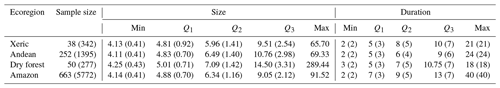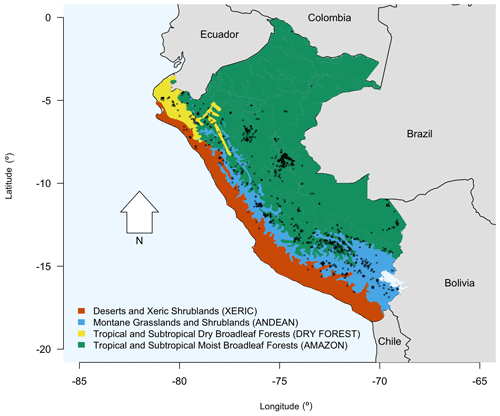Figure 2Map of the Peruvian ecoregions considered in this study, with 2001–2020 GlobFire centroids of large, multi-day fires overlaid.

Five fire danger indexes (FDIs) are calculated using ERA5 reanalysis for 2001–20202: the burning index (BI), energy release component (ERC), spread component (SC) , fire weather index (FWI), and Keetch–Byram drought index (KBDI) . The raw values were converted into a score by subtracting the mean and dividing by the standard deviation calculated from 2001–2020 data. Additionally, land cover data came from the GlobCover dataset 3. GlobCover data use satellite measurements to classify land cover globally into one of 22 categories at a 300 m resolution. GlobCover data were used to characterize the general composition of land cover within the burned area of wildfires.

The FDI values for each incident were extracted on the reported ignition date of the fire and at the incident centroid. The number of pixels within the final burn perimeter from each land cover type was recorded, reclassified using Table (2), and converted into percentages.

## 3.2 Estimation of model parameters

For each incident, the spread rate (r) and the extinguish rate (l) are estimated using the burned area time series derived from GlobFire. Specifically, for each burned area time series, the values of r and l that minimized the root mean square error (RMSE) were identified using the Nelder–Mead optimization algorithm . To increase the likelihood that the global optimum was identified, 1000 initial best guesses of the model parameters were obtained by using a normal distribution with a standard deviation of 1 to jitter the estimates produced with the methods described in Sect. 2.3. The optimization routine was run for each of the 1000 best guesses, and the run producing the lowest root mean square error was assumed to be the global optimum. The correlation of these two parameters was explored using two methods. Firstly, the spearman correlations of the spread rate and extinguish rate estimates were calculated for each ecoregion. Secondly, a quadratic model that predicted the extinguish rate from the spread rate was fit using least-squares regression.

## 3.3 Statistical modeling

An initial set of 24 generalized linear models were considered for each ecoregion and model parameter. The 24 candidates were produced by considering all linear combinations of FDIs and land cover covariates such that at most one variable from each variable category was used. The generalized linear model assumed an inverse link and gamma density function. The gamma distribution was selected because it, like the model parameters, is defined over a semi-infinite support and, when compared to the lognormal or inverse Gaussian distribution, provided a superior goodness of fit. The goodness of fit of the gamma density function4 was verified via visual inspection of QQ plots with a 95 % simultaneous confidence band (see Appendix A) and was calculated using the distrMod package . The inverse function was selected because it is the canonical link function for the gamma distribution (Faraway2016). The best models were selected using Akaike weights , which represent the probability that a model candidate was the true best-approximating model from the original set of 24 models. Akaike weights are calculated from a commonly used model selection criterion, Akaike information criterion (AIC), but have the added benefit of gauging the level of model uncertainty with probabilities, which are easier to interpret than the raw AIC values. The out-of-sample performance of the best-approximating models were estimate using a 3-fold cross validation. Each fire event for each region was placed into one of three folds according to the year in which the fire event occurred: 2000–2006, 2007–2013, and 2014–2020. Each fold was used as a test set once and used as a training set twice . The average RMSE (Eq. 15) and symmetrical mean absolute percent error (Eq. 16) were used to measure out-of-sample model performance. All calculations were performed in the R programming environment .

$\begin{array}{}\text{(15)}& \mathrm{RMSE}=\sqrt{\frac{\mathrm{1}}{n}\sum _{i=\mathrm{1}}^{n}\left({y}_{i}-{f}_{i}{\right)}^{\mathrm{2}}}\end{array}$

$\begin{array}{}\text{(16)}& \mathrm{sMAPE}=\frac{\mathrm{200}}{n}\sum _{i=\mathrm{1}}^{n}\frac{|{y}_{i}-{f}_{i}|}{{y}_{i}+{f}_{i}}\end{array}$

## 3.4 Sensitivity analysis

Because size-based filtering of wildfire events can effect the results of statistical analyses , the main analysis was also repeated without filtering and compared to the original analysis to describe the consequences of this methodological choice. Specifically, changes to the structure of the best-approximating model and changes in Akaike weights were identified.

4 Results

## 4.1 Difference equation parameters and performance

Overall, mean fire spread rates were approximately a 0.5 km d−1, but this quantity varied by ecoregion. The mean spread rates were highest in the Andean and dry forest ecoregions and were lowest in the Amazon ecoregion. The mean extinguish rates were usually near 2 km d−1, but like spread rates, this quantity varied by ecoregion. The mean extinguish rate was highest in the dry forest ecoregion and lowest in the Amazon ecoregion (Fig. 3). The gamma distribution provided a high-quality parametric approximation of the distribution of observed spread rates (Fig. A1) and a slightly lower quality approximation of the distribution of extinguish rates (Fig. A2).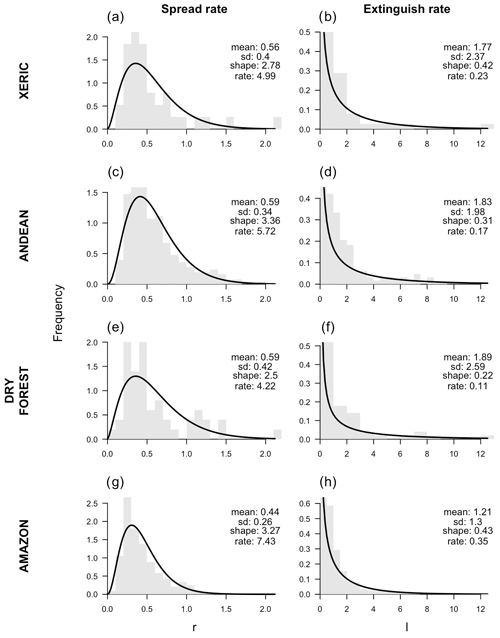Figure 3Histograms of the spread rates and extinguish rates (in kilometers per day) disaggregated by ecoregion. Maximum-likelihood estimates of the gamma distribution are overlaid. The mean, standard deviation, estimated shape, and estimated rate parameters are reported in the upper right of each panel.

The spread rate and extinguish rate were highly correlated with one another. Spearman correlation coefficients ranged from 0.91 in the dry forest ecoregion to 0.96 in the Amazon and xeric ecoregions. The observed relationship between the two parameters was well described using a quadratic model (Fig. 4).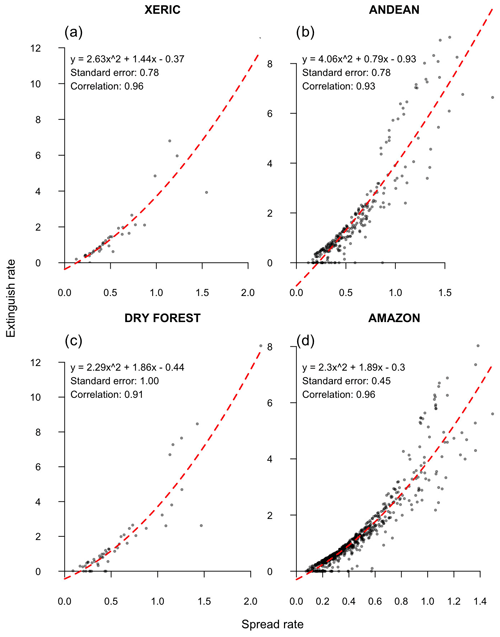Figure 4Scatterplots of the observed relationship between spread rate and extinguish rate (in kilometers) disaggregated by ecoregion. Least-squares fit of quadratic model and spearman correlation coefficient are reported in the upper left of each panel.

In both absolute and relative terms, the difference equations approximated the observed burned area time series across all ecoregions well. The lowest median RMSE (0.54 km2) was seen in xeric ecoregion, whereas the highest median RMSE was seen in dry forests (0.76 km2). Similarly, the lowest symmetric mean absolute percentage error (sMAPE) was observed in the xeric ecoregion (20.36 %), and the highest sMAPE was observed in the dry forests (23.25 %). In terms of RMSE, the difference equation model did particularly well on fires smaller than 10 km2 and fires less than 3 weeks in duration (Fig. 5). However, when sMAPE was instead considered, there was little difference in performance between small short-duration fires and large long-duration fires.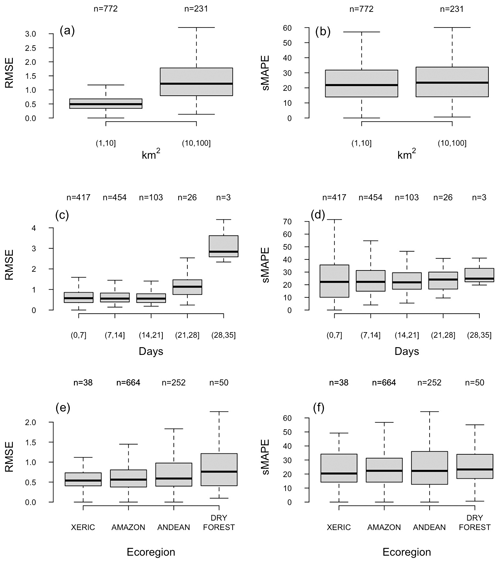Figure 5Boxplots of root-mean square error estimates calculated from best-fit difference equation predictions and GlobFire observations. Root-mean square error estimates are disaggregated by size, duration, and ecoregion. Sample size is reported above the boxplots, and outliers have been removed for visualization purposes.

## 4.2 Best-fitting parameter models

In the xeric ecoregion, the best-approximating model of spread rates predicted that fire would grow faster as FWI increased and as the percent of anthropogenic cover decreased, but the relationships were only weakly statistically significant. In the Andean ecoregion, a strongly significant positive relationship between fire spread rates and the SC was observed. The best-approximating spread model in the dry forest ecoregion did not include any FDI or land cover variables. In the Amazon ecoregion, the best-approximating model of spread assumed a positive relationship with SC and a positive relationship with percent grassland land cover. In the Amazon ecoregion, the effect of the SC on fire spread and the effect of grassland cover on fire spread were very strongly significant (Table 3).

Table 3Summary of spread parameter generalized linear models for each ecoregion. Summary including formula, Akaike weight, and significance level.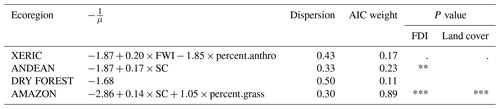P values greater than 0.10 are interpreted as not significant (ns), P values less than 0.1 are interpreted as weakly significant (.), P values less than 0.05 are interpreted as significant (*), P values less than 0.01 are interpreted as strongly significant (**), and P values less than 0.001 are interpreted as very strongly significant (***).

In the xeric and dry forest ecoregions, the best-approximating extinguish rate models did not include any covariates. In the Andean ecoregion, the best-approximating model reported a strongly significant and positive relationship between SC and the extinguish rate. In the Amazon ecoregion, extinguish rates were predicted to increase with the SC and percent grassland land cover; both covariates were very strongly significant (Table 4).

Table 4Summary of extinguish rate generalized linear models for each ecoregion. Summary including formula, Akaike weight, and significance level. P values greater than 0.10 are interpreted as not significant (ns), P values less than 0.1 are interpreted as weakly significant (.), P values less than 0.05 are interpreted as significant (*), P values less than 0.01 are interpreted as strongly significant (**), and P values less than 0.001 are interpreted as very strongly significant (***).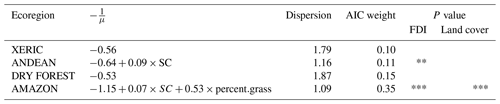For the spread models, the Akaike weights – the probability that the true best-approximating model was selected from the 24 candidates – ranged from 0.11 in the dry forest ecoregion to 0.89 in the Amazon ecoregion (Table 3). For the extinguish rate models, this probability ranged from 0.10 in the xeric ecoregion to 0.35 in the Amazon ecoregion (Table 4). The probability that the true best-approximating spread model contained both a land cover and FDI covariate ranged was 0.43 in the dry forest ecoregion, 0.54 in the Andean ecoregion, 0.68 in the xeric ecoregion, and >0.99 in the Amazon. The probability that the true best-approximating extinguish model contained both a land cover and FDI covariate was lower and ranged from 0.36 in the dry forest ecoregion, 0.45 in the Andean ecoregion, 0.47 in the xeric ecoregion, and 0.88 in the Amazon ecoregion (Fig. 6).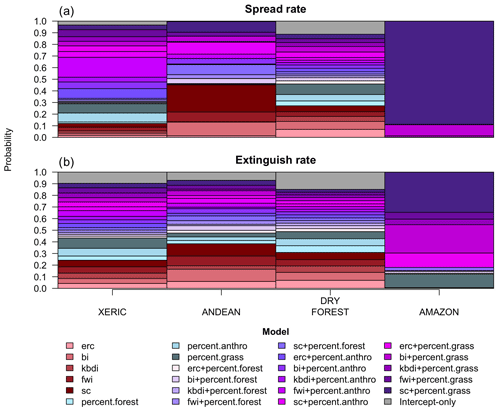Figure 6Bar plots of Akaike weights – the probability that each model candidate is truly the best-approximating model – for each ecoregion and parameter. Models that contain both an FDI and land cover covariate are colored purple, those that contain only a FDI are colored red, those that only contain a land cover variable are colored blue, and the intercept-only models are colored grey.

The spread rate estimates produced from the best-approximating generalized linear models were expected to get within about 0.3 to 0.4 of the best-fitting spread rate estimates, but this performance varied by ecoregion. The best model performance was seen in the Amazon ecoregion, and the worst model performance was seen in the dry forest ecoregion, but the variability in RMSE estimates was not high. Similar results were observed in terms of relative performance. The spread rate estimates produced from the best-approximating generalized linear model were expected to get within 40 % to 60 % of the true spread rates, and when considering the sMAPE, the best model performance was again observed in the Amazon ecoregion, and the worst model performance was observed in the dry forest ecoregion (Table 5)

Model performance was slightly less in the extinguish rate models than in the spread rate models. The best-approximating generalized linear models were expected to get within about 1.3 to 2.6 of the best-fitting spread rate estimates. The best model performance was observed in the Amazon ecoregion, and the worst model performance was observed in the dry forest ecoregion. Similar patterns were observed when considering the sMAPE. The best-approximating generalized linear models were expected to get within 70 % to 100 % of the true spread rates, with the best performance being observed in the Amazon ecoregion and the worst performance being observed in the dry forest ecoregion (Table 6).

Table 5Estimated performance of spread rate models as calculated from 3-fold cross validation. Each row reports the test years defining each fold.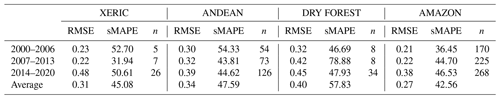Table 6Estimated performance of extinguish rate models as calculated from 3-fold cross validation. Each row reports the test years defining each fold.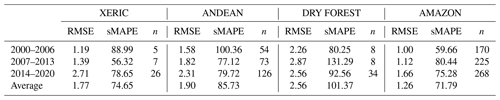The effects of fire weather and land cover varied dramatically across ecoregions. In the xeric ecoregion, large FWI values were characterized with noticeably larger, faster growing, and longer duration fires compared to small FWI values. High anthropogenic cover was associated with much smaller, slower spreading, and shorter duration fires compared to areas with low anthropogenic cover. Models that included fire weather and land cover had no detectable advantage over models that used no covariates at all in the dry forest ecoregions. In the Andean ecoregion, increases in the SC tended to result in slightly larger and faster growing fires, but the predicted fire duration was nominally smaller in the highest fire danger scenario considered. In the Amazon ecoregion, high SC values were characterized by increases in expected fire size and spread rate and slight decreases in fire duration. Increases in grassland cover were predicted to increase fire size and spread rates and decrease fire duration (Fig. 7).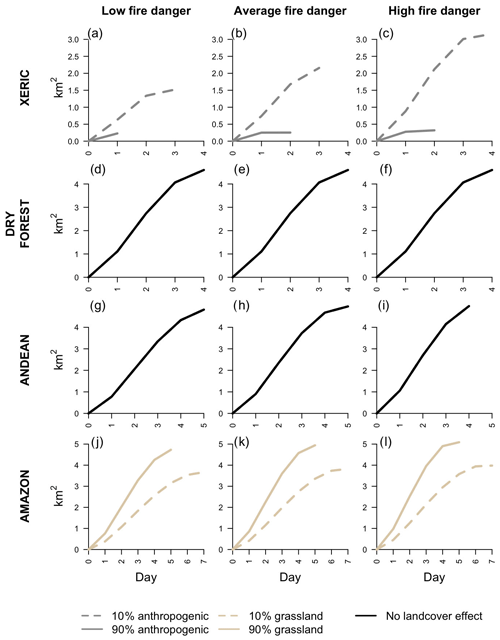Figure 7Expected daily burned area time series for each ecoregion under low fire danger (20th percentile of relevant FDI), normal fire danger (median value of the relevant FDI), and high fire danger (80th percentile of relevant FDI) conditions and at two extremes of the relevant land cover covariate.

## 4.3 Sensitivity analysis

Repeating the analysis without filtering could change the model structure and uncertainty for both the spread and extinguish models. When the size-based filtering was omitted from the model fitting procedure in the xeric ecoregion, the best-approximating spread model changed from one that used FWI and anthropogenic land cover as covariates to one that used the SC and grassland cover as covariates. In the Andean ecoregion, the same changes in methodology changed the best-approximating spread model from one the used the SC only to one that used the BI and grassland cover as covariates. In the dry forest, these changes in methodology resulted in a best-approximating spread model that used the burning index when previously no covariates were used in the best-approximating spread model. The most modest changes were observed in the Amazon ecoregion, where the same set of covariates were used regardless if filtering was applied or not, although the effect that these covariates had on spread rates were sensitive to these changes in methodology (Table 7).

Similarly, the structure of the extinguish rate models were also sensitive to the choice to apply size-based filtering. When the size-based filtering was applied, the best-approximating extinguish rate model in xeric and dry forest ecoregions used no covariates, but when the size-based filtering was not applied, the best-approximating models used the SC and BI, respectively. As with the spread models, in the Andean region the best-approximating model used the spread component when size-based filtering was used and used the BI and grassland cover when size-based filtering was not used. Lastly, the robustness to choices in filtering methodology that were observed in the spread models for the Amazon ecoregion were likewise observed when considering the extinguish rate models (Table 8).

Not only could the model structure change based on whether or not size-based filtering was applied but also the level of model uncertainty could be sensitive to this decision. Specifically, AIC weights of the best-approximating models tended to be much higher when the size-based filtering was not applied compared to when it was. In other words, the probability that the model that was identified as best truly was the best-approximating model was higher when size-based filtering was not used. The only exception to this trend was observed in the extinguish rate models for the dry forest ecoregion, where the AIC weight was 0.15 when size-based filtering was applied versus 0.11 when size-based filtering was not applied (Tables 78).

Table 7Summary of spread parameter generalized linear models for each ecoregion as fit without using size-based filtering. Summary including formula, Akaike weight, and significance level. P values greater than 0.10 are interpreted as not significant (ns), P values less than 0.1 are interpreted as weakly significant (.), P values less than 0.05 are interpreted as significant (*), P values less than 0.01 are interpreted as strongly significant (**), and P values less than 0.001 are interpreted as very strongly significant (***).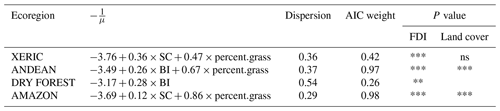Table 8Summary of extinguish parameter generalized linear models for each ecoregion as fit without using size-based filtering. Summary including formula, Akaike weight, and significance level. P values greater than 0.10 are interpreted as not significant (ns), P values less than 0.1 are interpreted as weakly significant (.), P values less than 0.05 are interpreted as significant (*), P values less than 0.01 are interpreted as strongly significant (**), and P values less than 0.001 are interpreted as very strongly significant (***).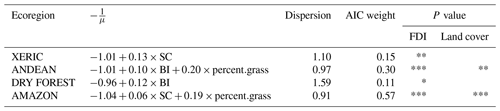5 Discussion

## 5.1 Difference equation validity

All models are approximations of the real world, and the relevant question to ask is whether the models can provide useful information . With this in mind, we argue that the difference equation model presented in this paper has a number of advantages over other approaches used to model fire growth. Firstly, the model is simple, requiring estimation of only a few parameters. The difference equation model is therefore vastly easier to implement than other commonly used spread models that require complex data inputs and subjective decisions regarding initialization parameters (Sullivan2009b, a). The model parameters are also easily interpreted and are directly related to the underlying fire processes. Although alternative difference equation models exist that could adequately represent observed fire growth , the interpretation of the model parameters in these alternative models is cumbersome because they were not originally developed to represent fire growth.

## 5.2 Effects of meteorology and land cover on fire development

In aggregate, the spread rate and extinguish rate models produce fire progressions that are plausible. In all but the dry forest region, increases in FDIs produce larger and faster growing fires. Moreover, the effects of land cover are likewise believable. In the xeric ecoregion it would make sense that – given the destructive effects of fire to human health and economic activity – human presence would have an inhibitory effect on fire. Similarly, given that forest vegetation can inhibit fire growth , it is reasonable to predict larger and faster growing fires in grassland cover. The negative relationship between FDIs and duration should not be accepted uncritically, although potential explanations could exist. In fragmented landscapes, it is conceivable that fire size is constrained by available fuels and that increased fire danger merely hastens the consumption of these fuels. That is, fire size may be fixed due to fuel discontinuity, and increased fire danger just hastens the inevitable outcome in these circumstances. Still, fragmentation is likely an unsatisfactory explanation for much of Peru, and like the relationship between extinguish rates and FDIs, we should also not discount the possibility that the predicted relationship between fire duration and FDIs might be better explained as a model artifact rather than a real-world causal relationship.

## 5.3 Future work

The results and methods in this paper provide a template for forecasting fire growth from environmental covariates. When a fire ignition is reported, estimates of the spread rate and extinguish rate can be calculated from environmental data, which would permit estimates of risk. Because the spatial domain of the GlobFire data covers the entire world, the methods described here can be readily applied to other ecoregions, which would be particularly useful to locations without access to sophisticated fire spread modeling capabilities. Although predictions from these models describe a time series of expected fire growth conditional on meteorological and land cover conditions, it is often the statistical extremes that are the most destructive and dangerous . These extreme events often occur in unique conditions , and their relative position in the statistical distribution of fire sizes means that these events are some of the hardest to predict (Fig. 5). For this reason, future work should further validate the predictive performance of these models to determine what additional information and methods are required to best predict the times when these extreme fires occur.

Future work might include additional covariates into these models to further improve predictive performance and by extension improve fire-related decisions. In addition to those explored in this paper, there are numerous other FDIs that could be included that might better model fire spread . Firefighting-related covariates could be used to forecast the effects various suppression strategies would have on fire behavior, and various ocean temperature indexes could be included to account for ENSO-related effects on weather that modulate fire spread . Forest structure and composition is a highly complex and important variable to consider when estimating fire growth but was not thoroughly investigated in this analysis for practical purposes. A South American forest containing native species versus one that is dominated by eucalyptus might burn much differently even though both could be characterized as forested . Projected climatic and land use changes could be used to provide longer-term forecasts of fire risk and inform decisions such as firefighting staffing and training programs, as well as inform land management decisions. The spread rates and extinguish rates would clearly differ between planned and unplanned fires, but differentiating between these two types of fires was not attempted in this study due to the difficulty in making this categorization from satellite data.

Given the high covariance of the two difference equation parameters and sometimes counterintuitive results, future work should also explore the necessity of forecasting extinguish rates from environmental data or if the extinguish rates may simply be inferred from predicted spread rates instead (Fig. 4). The model inputs are subject to uncertainty, and future work might investigate what the consequences of this uncertainty might be. For example, land cover estimates change over time, and areas that were once forested may become unforested, and vice versa. Hence, it is possible that some of the land cover data are corrupted, and crosschecking the results using other datasets can ensure that the results are robust. Similarly, although using meteorological information at the ignition date was a methodological convenience, it may not be representative of the conditions that occurred during the largest growth days. Although we determined that the level of temporal autocorrelation in the FDIs was reasonably high enough to justify using data at the ignition date, this uncertainty in the data inputs should be investigated further. We would like to note that performance was best in the Amazon, where most of the data were available, and performance was most variable in the ecoregions with the least data. We further note here that more complex models were permitted when size-based filtering was not performed. Both of these result suggest a need for continued data collection, so that sufficient amounts of data are available to produce reliable estimates of performance and that important relationships between fire and the environment are able to be identified.

6 Conclusions

In this paper, we developed a simple and intuitive difference equation model of fire growth that can be estimated using globally available satellite data. The difference equation was based on two parameters: the spread rate and the extinguish rate. We described the statistical distribution of these two parameters for four ecoregions in Peru using gamma distributions. We also built generalized linear models to predict these parameters using FDI and land cover as covariates. We found that FDIs – specifically SC, FWI, and BI – were useful predictors of fire spread and extinguishment in Peru. In general, when FDIs were used to predict these two parameters, we found that the SC was usually the best choice. However, model uncertainty was frequently high, and the identification of which FDI was best was sensitive to methodological choices regarding size-based filtering of fires. Compared to other regions, model confidence was particularly high in the Amazon ecoregion and low in the dry forest ecoregion. In addition to FDIs, land cover was also a useful predictor in some contexts. Specifically, anthropogenic cover had a weakly significant and negative effect on spread rates in the xeric ecoregion, and grassland cover had a positive and often very strongly significant effect on spread and extinguish rates in several contexts. Counterintuitive relationships were observed between extinguish rates and FDIs, where increased fire danger increased extinguish rates. These relationships were explained by the fairly strong correlation of the two model parameters and were concluded to be model artifacts rather than representing real-world causal relationships. We argue that the methods presented here, although simple and able to be improved upon, are a promising method of forecasting fire spread risk when sophisticated fire modeling capabilities are unavailable or impractical.

Appendix A:QQ plots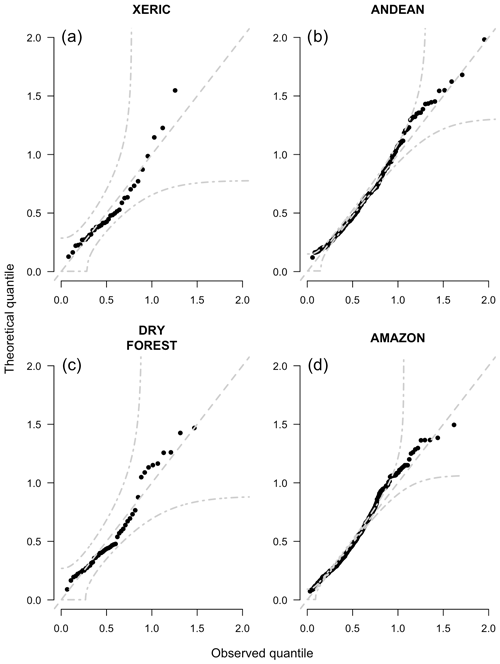Figure A1QQ plots of spread rate parameters disaggregated by ecoregion. The dashed lines show the 95 % and median simultaneous confidence band.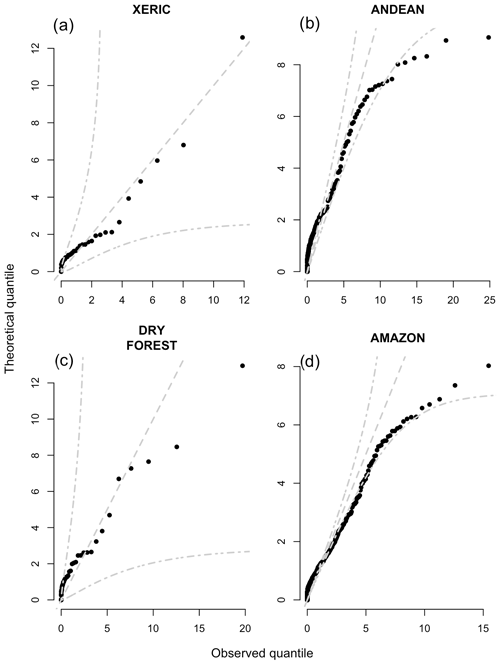Figure A2QQ plots of extinguish rate parameters disaggregated by ecoregion. The dashed lines show the 95 % and median simultaneous confidence band.

Code availability
Data availability

Fire progression data comes from the GlobFire Project which were created by and are available at https://doi.org/10.1038/s41597-019-0312-2. Terrestrial Ecoregion boundaries were produced by the 2009 Nature Conservancy Assessment and are available at https://geospatial.tnc.org/datasets/b1636d640ede4d6ca8f5e369f2dc368b/ (last access: 17 June 2022; ). Global Land Cover data for 2009 come from the European Space Agency and the Université Catholique de Louvain and are available at http://due.esrin.esa.int/page_globcover.php (last access: 20 May 2022; ). Fire weather data was produced William Matt Jolly in 2021 and can be downloaded at: https://www.wfas.net/data/SAR/ (last access: 29 April 2022).

Author contributions

Conceptualization, HRP; methodology, HRP and WMJ; software, HRP and AM; validation, HRP and WMJ; formal analysis, HRP and AM; investigation, HRP; resources, EA and WMJ; data curation, WMJ and AM; writing – original draft preparation, HRP; writing – review and editing, HRP, WMJ, EA, AM, SV, SBR, CTC, and BPV; visualization, HRP and SBR; supervision, WMJ and EA; project administration, HRP, BPV, WMJ, and EA; funding acquisition, WMJ and EA.

Competing interests

The contact author has declared that none of the authors has any competing interests.

Disclaimer

Publisher’s note: Copernicus Publications remains neutral with regard to jurisdictional claims in published maps and institutional affiliations.

Special issue statement

This article is part of the special issue “The role of fire in the Earth system: understanding interactions with the land, atmosphere, and society (ESD/ACP/BG/GMD/NHESS inter-journal SI)”. It is a result of the EGU General Assembly 2020, 4–8 May 2020.

Acknowledgements

The authors would like to thank Isidoro Solis, Vannia Aliaga-Nestares, and Diego Rodriguez-Zimmermann.

Financial support

This research was funded in part by the US Forest Service International Programs, the United States Agency for International Development, the USFS Rocky Mountain Research Station, the University of Washington, and the Fulbright Scholars Program.

Review statement

This paper was edited by Kirsten Thonicke and reviewed by three anonymous referees.

References

All, J., Medler, M., Arques, S., Cole, R., Woodall, T., King, J., Yan, J., and Schmitt, C.: Fire response to local climate variability: Huascarán National Park, Peru, Fire Ecol., 13, 85–104, 2017. a

Andela, N., Morton, D. C., Giglio, L., Paugam, R., Chen, Y., Hantson, S., van der Werf, G. R., and Randerson, J. T.: The Global Fire Atlas of individual fire size, duration, speed and direction, Earth Syst. Sci. Data, 11, 529–552, https://doi.org/10.5194/essd-11-529-2019, 2019. a, b, c, d, e, f

Anderson, D. and Burnham, K.: Model selection and multi-model inference, Second, NY: Springer-Verlag, 63, 10, ISBN 0-387-95364-7, 2004. a

Anderson, K. R., Englefield, P., Little, J., and Reuter, G.: An approach to operational forest fire growth predictions for Canada, Int. J. Wildland Fire, 18, 893–905, 2009. a

Arino, O., Gross, D., Ranera, F., Leroy, M., Bicheron, P., Brockman, C., Defourny, P., Vancutsem, C., Achard, F., Durieux, L., et al.: GlobCover: ESA service for global land cover from MERIS, in: 2007 IEEE international geoscience and remote sensing symposium, 2412–2415, IEEE, https://doi.org/10.1109/igarss.2007.4423328, 2007. a

Artés, T., Oom, D., De Rigo, D., Durrant, T. H., Maianti, P., Libertà, G., and San-Miguel-Ayanz, J.: A global wildfire dataset for the analysis of fire regimes and fire behaviour, Sci. Data [data set], ISSN 2052-4463, 6, JRC116346, https://doi.org/10.1038/s41597-019-0312-2, 2019. a, b

Barbero, R., Abatzoglou, J. T., Kolden, C. A., Hegewisch, K. C., Larkin, N. K., and Podschwit, H.: Multi-scalar influence of weather and climate on very large-fires in the Eastern United States, Int. J. Climatol., 35, 2180–2186, 2015a. a

Barbero, R., Abatzoglou, J. T., Larkin, N. K., Kolden, C. A., and Stocks, B.: Climate change presents increased potential for very large fires in the contiguous United States, Int. J. Wildland Fire, 24, 892–899, 2015b. a

Bistinas, I., Oom, D., Sá, A. C., Harrison, S. P., Prentice, I. C., and Pereira, J. M.: Relationships between human population density and burned area at continental and global scales, PLOS ONE, 8, e81188, https://doi.org/10.1371/journal.pone.0081188, 2013. a

Block, M. and Richter, M.: Impacts of heavy rainfalls in El Niño 1997/98 on the vegetation of Sechura Desert in Northern Peru (a preliminary report), Phytocoenologia, 30, 491–517, 2000. a, b

Bradshaw, L. S., Deeming, J. E., Burgan, R. E., and Cohen, J. D.: The 1978 National Fire-Danger Rating System: technical documentation, General Technical Report INT-169, U.S. Department of Agriculture, Forest Service, Intermountain Forest and Range Experiment Station., Ogden, UT, https://www.fs.fed.us/rm/pubs_int/int_gtr169.pdf (last access: 16 July 2023), 1984. a, b, c, d, e, f

Bufacchi, P., Santos, J. C., Veras, C. A. G., Alvarado, E. C., Mell, W., Carvalho, J. A., Filho, G., Krieger, C., Bufacchi, P., Santos, J. C., Gurgel Veras, C. A., Alvarado, E. C., Mell, W., and Carvalho, J. A.: Probability of surface fire spread in Brazilian rainforest fuels from outdoor experimental measurements, Eur. J. For. Res., 136, 217–232, 2017. a, b, c

Butler, B. W.: Wildland firefighter safety zones: a review of past science and summary of future needs, Int. J. Wildland Fire, 23, 295–308, 2014. a

Chen, Y., Randerson, J. T., Morton, D. C., DeFries, R. S., Collatz, G. J., Kasibhatla, P. S., Giglio, L., Jin, Y., and Marlier, M. E.: Forecasting fire season severity in South America using sea surface temperature anomalies, Science, 334, 787–791, 2011. a

Cochrane, M. A.: Fire science for rainforests, Nature, 421, 913–919, 2003. a, b, c, d, e, f, g, h

Cruz, M. G., Cheney, N. P., Gould, J. S., McCaw, W. L., Kilinc, M., and Sullivan, A. L.: An empirical-based model for predicting the forward spread rate of wildfires in eucalypt forests, Int. J. Wildland Fire, 31, 81–95, 2021. a

Cullen, A. C., Axe, T., and Podschwit, H.: High-severity wildfire potential–associating meteorology, climate, resource demand and wildfire activity with preparedness levels, Int. J. Wildland Fire, 30, 30–41, 2020. a

De Vivo, F., Battipede, M., and Johnson, E.: Infra-red line camera data-driven edge detector in UAV forest fire monitoring, Aerosp. Sci. Technol., 111, 106574, https://doi.org/10.1016/j.ast.2021.106574, 2021. a

Deeming, J. E., Burgan, R. E., and Cohen, J. D.: The national fire-danger rating system, 1978, Vol. 39, Department of Agriculture, Forest Service, Intermountain Forest and Range Experiment Station, FRAMES no. 13899, 1977. a

Doerr, S. H. and Santín, C.: Global trends in wildfire and its impacts: perceptions versus realities in a changing world, Philos. T. R. Soc. B, 371, 20150345, https://doi.org/10.1098/rstb.2015.0345, 2016. a

ESA: GlobCover, Welcome to the European Space Agency GlobCover Portal, ESA [data set], http://due.esrin.esa.int/page_globcover.php (last access: 20 May 2022), 2010. a

Faraway, J. J.: Extending the linear model with R: generalized linear, mixed effects and nonparametric regression models, Chapman and Hall/CRC, ISBN 978-1584884248, 312 pp., 2016. a

Finney, M., Grenfell, I. C., and McHugh, C. W.: Modeling containment of large wildfires using generalized linear mixed-model analysis, Forest Sci., 55, 249–255, 2009. a

Fried, J. S. and Fried, B. D.: Simulating wildfire containment with realistic tactics, Forest Sci., 42, 267–281, 1996. a

Fujioka, F. M., Gill, A. M., Viegas, D. X., and Wotton, B. M.: Fire danger and fire behavior modeling systems in Australia, Europe, and North America, Dev. Environ. Sci., 8, 471–497, 2008. a

Galizia, L. F., Alcasena, F., Prata, G., and Rodrigues, M.: Assessing expected economic losses from wildfires in eucalypt plantations of western Brazil, Forest Policy Econ., 125, 102405, https://doi.org/10.1016/j.forpol.2021.102405, 2021. a

Hastie, T., Tibshirani, R., Friedman, J. H., and Friedman, J. H.: The elements of statistical learning: data mining, inference, and prediction, Vol. 2, Springer, ISBN 978-0387848570, 767 pp., 2009. a

Houtman, R. M., Montgomery, C. A., Gagnon, A. R., Calkin, D. E., Dietterich, T. G., McGregor, S., and Crowley, M.: Allowing a wildfire to burn: estimating the effect on future fire suppression costs, Int. J. Wildland Fire, 22, 871–882, 2013. a

Jolly, W. M., Cochrane, M. A., Freeborn, P. H., Holden, Z. A., Brown, T. J., Williamson, G. J., and Bowman, D. M.: Climate-induced variations in global wildfire danger from 1979 to 2013, Nat. Commun., 6, 1–11, 2015. a

Jolly, W. M., Freeborn, P. H., Page, W. G., and Butler, B. W.: Severe fire danger index: A forecastable metric to inform firefighter and community wildfire risk management, Fire, 2, 47, https://doi.org/10.3390/fire2030047, 2019. a, b, c

Keetch, J. J. and Byram, G. M.: A drought index for forest fire control, vol. 38, US Department of Agriculture, Forest Service, Southeastern Forest Experiment, 1968. a

Kolden, C. A. and Weisberg, P. J.: Assessing accuracy of manually-mapped wildfire perimeters in topographically dissected areas, Fire Ecol., 3, 22–31, 2007. a, b

Lima, A., Silva, T. S. F., de Feitas, R. M., Adami, M., Formaggio, A. R., and Shimabukuro, Y. E.: Land use and land cover changes determine the spatial relationship between fire and deforestation in the Brazilian Amazon, Appl. Geogr., 34, 239–246, 2012. a, b

Littell, J. S., Peterson, D. L., Riley, K. L., Liu, Y., and Luce, C. H.: A review of the relationships between drought and forest fire in the United States, Global Change Biol., 22, 2353–2369, 2016. a, b, c

Massman, W. J., Forthofer, J., and Finney, M. A.: An improved canopy wind model for predicting wind adjustment factors and wildland fire behavior, Can. J, Forest Res., 47, 594–603, 2017. a, b, c, d

Melcher, T., Zinke, R., Trott, M., and Krause, U.: Experimental investigations on the repeatability of real scale fire tests, Fire Safety J., 82, 101–114, 2016. a

Meyn, A., White, P. S., Buhk, C., and Jentsch, A.: Environmental drivers of large, infrequent wildfires: the emerging conceptual model, Prog. Phys. Geog., 31, 287–312, 2007. a, b

Nelder, J. A. and Mead, R.: A simplex method for function minimization, Comput. J., 7, 308–313, 1965. a

Page, W. G., Freeborn, P. H., Butler, B. W., and Jolly, W. M.: A review of US wildland firefighter entrapments: trends, important environmental factors and research needs, Int. J. Wildland Fire, 28, 551–569, 2019. a, b

Podschwit, H.: CURATE_REGIONAL_GLOBFIRE_DATASET.R, figshare, Software [code], https://doi.org/10.6084/m9.figshare.23674185.v1, 2023a. a

Podschwit, H.: NHESS_PAPER_CODE.R, figshare, Software [code], https://doi.org/10.6084/m9.figshare.23673306.v1, 2023b. a

Podschwit, H.: WILDFIRE_GROWTH_FUNCTIONS.R, figshare, Software [code], https://doi.org/10.6084/m9.figshare.23673393.v1, 2023c. a

Podschwit, H.: DERIVE_BATS_DATASET_FROM_GLOBFIRE.R, figshare, Software [code], https://doi.org/10.6084/m9.figshare.23673465.v1, 2023d. a

Podschwit, H. and Cullen, A.: Patterns and trends in simultaneous wildfire activity in the United States from 1984 to 2015, Int. J. Wildland Fire, 29, 1057–1071, 2020. a

Podschwit, H., Guttorp, P., Larkin, N., and Steel, E. A.: Estimating wildfire growth from noisy and incomplete incident data using a state space model, Environ. Ecol. Stat., 25, 325–340, 2018. a, b, c

Podschwit, H., Jolly, W., Alvarado, E., Verma, S., Ponce, B., Markos, A., Aliaga-Nestares, V., and Rodriguez-Zimmermann, D.: Reliability of cross-regional applications of global fire danger models: a Peruvian case study, Fire Ecol., 18, 25, https://doi.org/10.1186/s42408-022-00150-7, 2022. a

Price, O. F., Borah, R., and Maier, S. W.: Role of weather and fuel in stopping fire spread in tropical savannas, Austral. Ecol., 39, 135–144, 2014. a

R Core Team: R: A language and environment for statistical computing, Vienna: R Core Team, 2013. a, b

Rapp, C. E., Wilson, R. S., Toman, E. L., and Jolly, W. M.: Assessing the role of short-term weather forecasts in fire manager tactical decision-making: a choice experiment, Fire Ecol., 17, 1–17, 2021. a, b

Ray, D., Nepstad, D., and Moutinho, P.: Micrometeorological and canopy controls of fire susceptibility in a forested Amazon landscape, Ecol. Appl., 15, 1664–1678, 2005. a, b, c, d, e

Reed, W. J. and McKelvey, K. S.: Power-law behaviour and parametric models for the size-distribution of forest fires, Ecol. Model, 150, 239–254, 2002. a, b

Short, K. C.: A spatial database of wildfires in the United States, 1992-2011, Earth Syst. Sci. Data, 6, 1–27, https://doi.org/10.5194/essd-6-1-2014, 2014. a

Slocum, M. G., Beckage, B., Platt, W. J., Orzell, S. L., and Taylor, W.: Effect of climate on wildfire size: a cross-scale analysis, Ecosystems, 13, 828–840, 2010. a

Stephens, S. L., Burrows, N., Buyantuyev, A., Gray, R. W., Keane, R. E., Kubian, R., Liu, S., Seijo, F., Shu, L., Tolhurst, K. G., et al.: Temperate and boreal forest mega-fires: Characteristics and challenges, Front. Ecol. Environ., 12, 115–122, 2014. a, b

Sullivan, A. L.: Wildland surface fire spread modelling, 1990–2007. 2: Empirical and quasi-empirical models, Int. J. Wildland Fire, 18, 369–386, 2009a. a, b

Sullivan, A. L.: Wildland surface fire spread modelling, 1990–2007. 1: Physical and quasi-physical models, Int. J. Wildland Fire, 18, 349–368, 2009b. a, b

Syphard, A. D., Keeley, J. E., Pfaff, A. H., and Ferschweiler, K.: Human presence diminishes the importance of climate in driving fire activity across the United States, P. Natl. Acad. Sci.-Biol., 114, 13750–13755, 2017.  a

Taylor, S. W., Woolford, D. G., Dean, C., and Martell, D. L.: Wildfire prediction to inform fire management: statistical science challenges, Stat. Sci., 28, 586–615, 2013. a

The Nature Conservancy: Terrestrial Ecoregions, The Nature Conservancy [data set], https://geospatial.tnc.org/datasets/b1636d640ede4d6ca8f5e369f2dc368b/ (last access: 17 June 2022), 2019. a

Twidwell, D., Wonkka, C. L., Sindelar, M. T., and Weir, J. R.: First approximations of prescribed fire risks relative to other management techniques used on private lands, PLOS ONE, 10, e0140410, https://doi.org/10.1371/journal.pone.0140410, 2015. a

Van Wagner, C. E.: Structure of the Canadian forest fire weather index, vol. 1333, Environment Canada, Forestry Service Ontario, 1974. a

Viegas, D. X. and Simeoni, A.: Eruptive behaviour of forest fires, Fire Technol., 47, 303–320, 2011. a, b

Wagenmakers, E.-J. and Farrell, S.: AIC model selection using Akaike weights, Psychon. B. Rev., 11, 192–196, 2004. a

Wang, X., Parisien, M.-A., Taylor, S. W., Candau, J.-N., Stralberg, D., Marshall, G. A., Little, J. M., and Flannigan, M. D.: Projected changes in daily fire spread across Canada over the next century, Environ. Res. Lett., 12, 025005, https://doi.org/10.1088/1748-9326/aa5835, 2017. a

Wei, Y., Thompson, M. P., Belval, E., Gannon, B., Calkin, D. E., and O'Connor, C. D.: Comparing contingency fire containment strategies using simulated random scenarios, Nat. Resour. Model, 34, e12295, https://doi.org/10.1111/nrm.12295, 2021. a

Zhong, X., Duckham, M., Chong, D., and Tolhurst, K.: Real-time estimation of wildfire perimeters from curated crowdsourcing, Sci. Rep.-UK, 6, 1–10, https://doi.org/10.1038/srep24206, 2016. a

https://www.wfas.net/data/SAR/, last access: 29 April 2022

QQ plots of the lognormal and inverse gamma distribution were omitted to keep the paper concise but can be requested from the corresponding author.